Diagnostic plots for the No-U-Turn-Sampler (NUTS), the default MCMC algorithm used by Stan. See the Plot Descriptions section, below.

mcmc_nuts_acceptance(x, lp, chain = NULL, ..., binwidth = NULL)

mcmc_nuts_divergence(x, lp, chain = NULL, ...)

mcmc_nuts_stepsize(x, lp, chain = NULL, ...)

mcmc_nuts_treedepth(x, lp, chain = NULL, ...)

mcmc_nuts_energy(x, ..., binwidth = NULL, alpha = 0.5, merge_chains = FALSE)

## Arguments

x A molten data frame of NUTS sampler parameters, either created by nuts_params() or in the same form as the object returned by nuts_params(). A molten data frame of draws of the log-posterior or, more commonly, of a quantity equal to the log-posterior up to a constant. lp should either be created via log_posterior() or be an object with the same form as the object returned by log_posterior(). A positive integer for selecting a particular chain. The default (NULL) is to merge the chains before plotting. If chain = k then the plot for chain k is overlaid (in a darker shade but with transparency) on top of the plot for all chains. The chain argument is not used by mcmc_nuts_energy(). Currently ignored. An optional value passed to ggplot2::geom_histogram() to override the default binwidth. For mcmc_nuts_energy() only, the transparency (alpha) level in [0,1] used for the overlaid histogram. For mcmc_nuts_energy() only, should all chains be merged or displayed separately? The default is FALSE, i.e., to show the chains separately.

## Value

A gtable object (the result of calling gridExtra::arrangeGrob()) created from several ggplot objects, except for mcmc_nuts_energy(), which returns a ggplot object.

## Quick Definitions

For more details see Stan Development Team (2016) and Betancourt (2017).

• accept_stat__: the average acceptance probabilities of all possible samples in the proposed tree.

• divergent__: the number of leapfrog transitions with diverging error. Because NUTS terminates at the first divergence this will be either 0 or 1 for each iteration.

• stepsize__: the step size used by NUTS in its Hamiltonian simulation.

• treedepth__: the depth of tree used by NUTS, which is the log (base 2) of the number of leapfrog steps taken during the Hamiltonian simulation.

• energy__: the value of the Hamiltonian (up to an additive constant) at each iteration.

## Plot Descriptions

mcmc_nuts_acceptance()

Three plots:

• Histogram of accept_stat__ with vertical lines indicating the mean (solid line) and median (dashed line).

• Histogram of lp__ with vertical lines indicating the mean (solid line) and median (dashed line).

• Scatterplot of accept_stat__ vs lp__.

mcmc_nuts_divergence()

Two plots:

• Violin plots of lp__|divergent__=1 and lp__|divergent__=0.

• Violin plots of accept_stat__|divergent__=1 and accept_stat__|divergent__=0.

mcmc_nuts_stepsize()

Two plots:

• Violin plots of lp__ by chain ordered by stepsize__ value.

• Violin plots of accept_stat__ by chain ordered by stepsize__ value.

mcmc_nuts_treedepth()

Three plots:

• Violin plots of lp__ by value of treedepth__.

• Violin plots of accept_stat__ by value of treedepth__.

• Histogram of treedepth__.

mcmc_nuts_energy()

Overlaid histograms showing energy__ vs the change in energy__. See Betancourt (2016) for details.

Betancourt, M. (2017). A conceptual introduction to Hamiltonian Monte Carlo. https://arxiv.org/abs/1701.02434

Betancourt, M. and Girolami, M. (2013). Hamiltonian Monte Carlo for hierarchical models. https://arxiv.org/abs/1312.0906

Hoffman, M. D. and Gelman, A. (2014). The No-U-Turn Sampler: adaptively setting path lengths in Hamiltonian Monte Carlo. Journal of Machine Learning Research. 15:1593--1623.

Stan Development Team. Stan Modeling Language Users Guide and Reference Manual. https://mc-stan.org/users/documentation/

## See also

• The Visual MCMC Diagnostics vignette.

• Several other plotting functions are not NUTS-specific but take optional extra arguments if the model was fit using NUTS:

• mcmc_trace(): show divergences as tick marks below the trace plot.

• mcmc_parcoord(): change the color/size/transparency of lines corresponding to divergences.

• mcmc_scatter(): change the color/size/shape of points corresponding to divergences.

• mcmc_pairs(): change the color/size/shape of points corresponding divergences and/or max treedepth saturation.

Other MCMC: MCMC-combos, MCMC-diagnostics, MCMC-distributions, MCMC-intervals, MCMC-overview, MCMC-parcoord, MCMC-recover, MCMC-scatterplots, MCMC-traces

## Examples

# \dontrun{
library(ggplot2)
library(rstanarm)
fit <- stan_glm(mpg ~ wt + am, data = mtcars, iter = 1000, refresh = 0)
np <- nuts_params(fit)
lp <- log_posterior(fit)

color_scheme_set("brightblue")
mcmc_nuts_acceptance(np, lp)#> stat_bin() using bins = 30. Pick better value with binwidth.mcmc_nuts_acceptance(np, lp, chain = 2)#> stat_bin() using bins = 30. Pick better value with binwidth.#> stat_bin() using bins = 30. Pick better value with binwidth.mcmc_nuts_divergence(np, lp)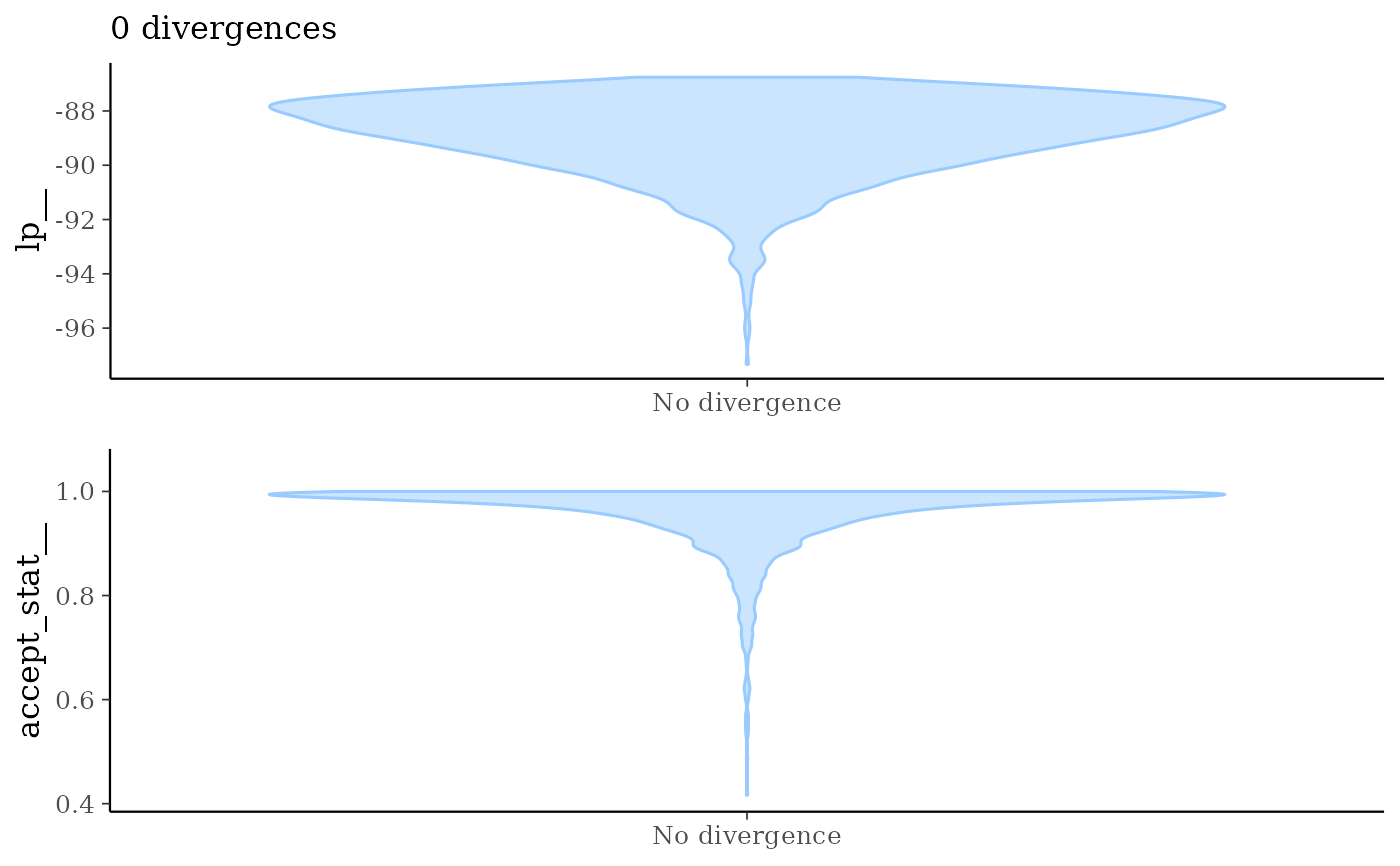mcmc_nuts_stepsize(np, lp)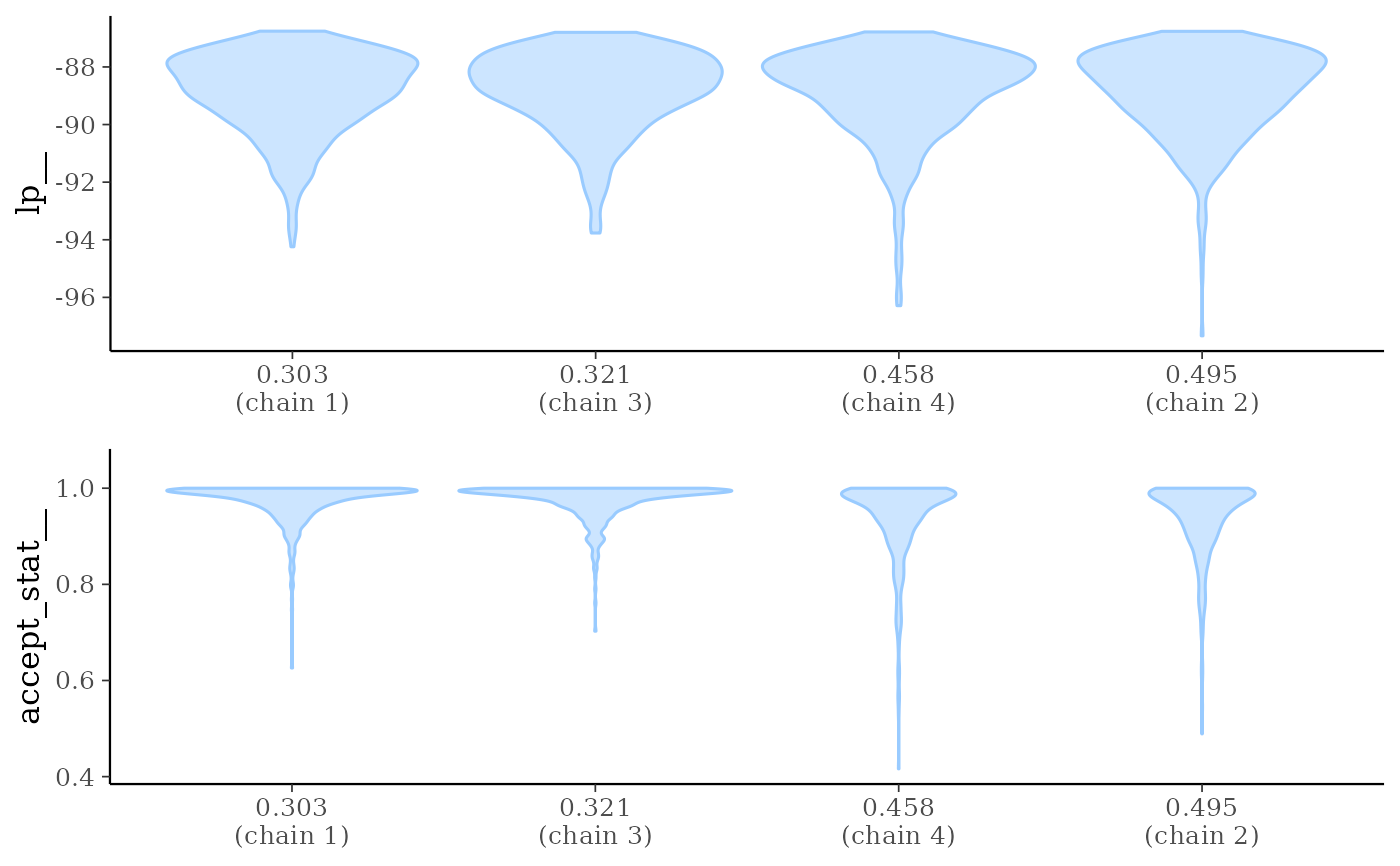mcmc_nuts_treedepth(np, lp)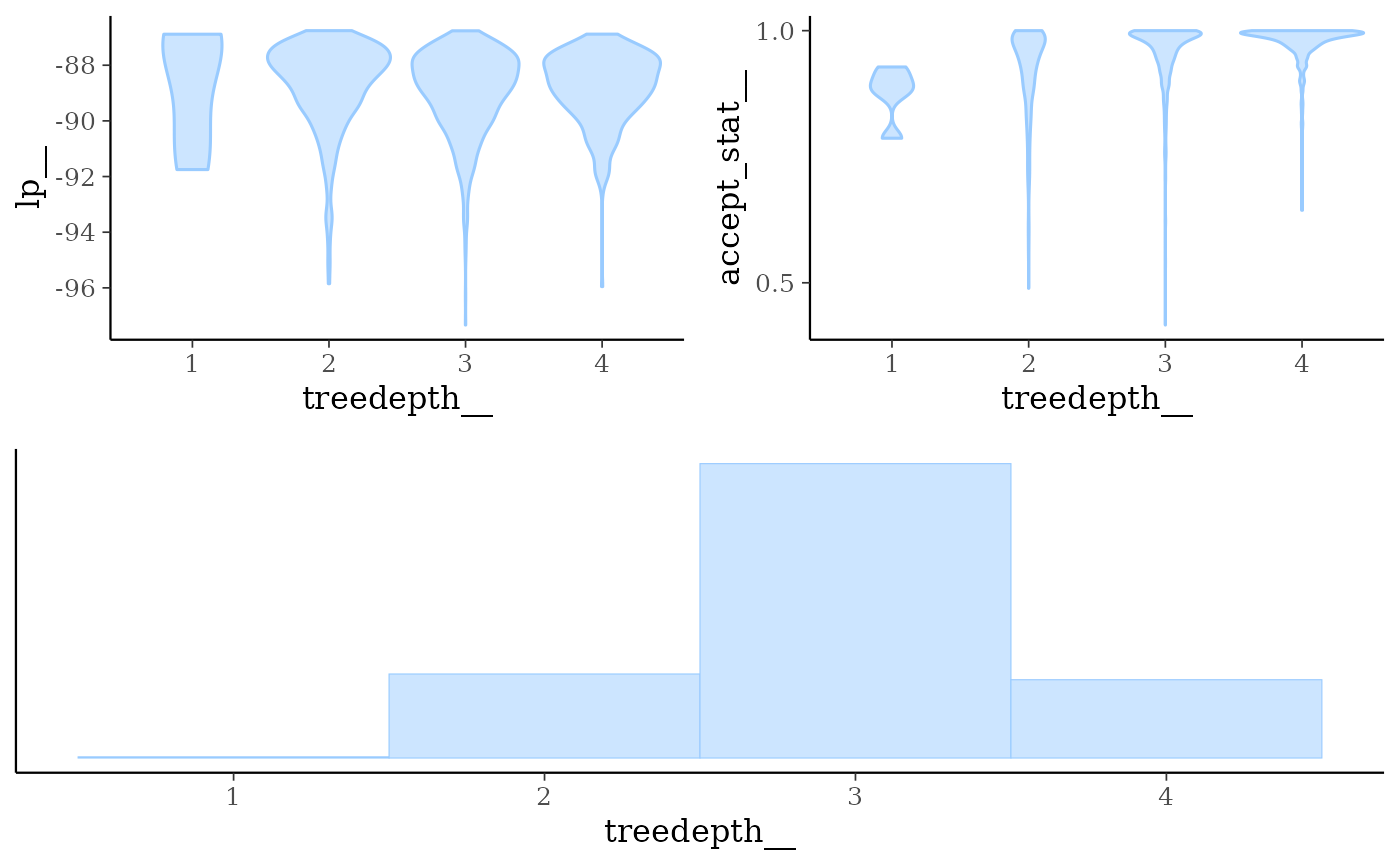color_scheme_set("red")
mcmc_nuts_energy(np)#> stat_bin() using bins = 30. Pick better value with binwidth.#> stat_bin() using bins = 30. Pick better value with binwidth.mcmc_nuts_energy(np, merge_chains = TRUE, binwidth = .15)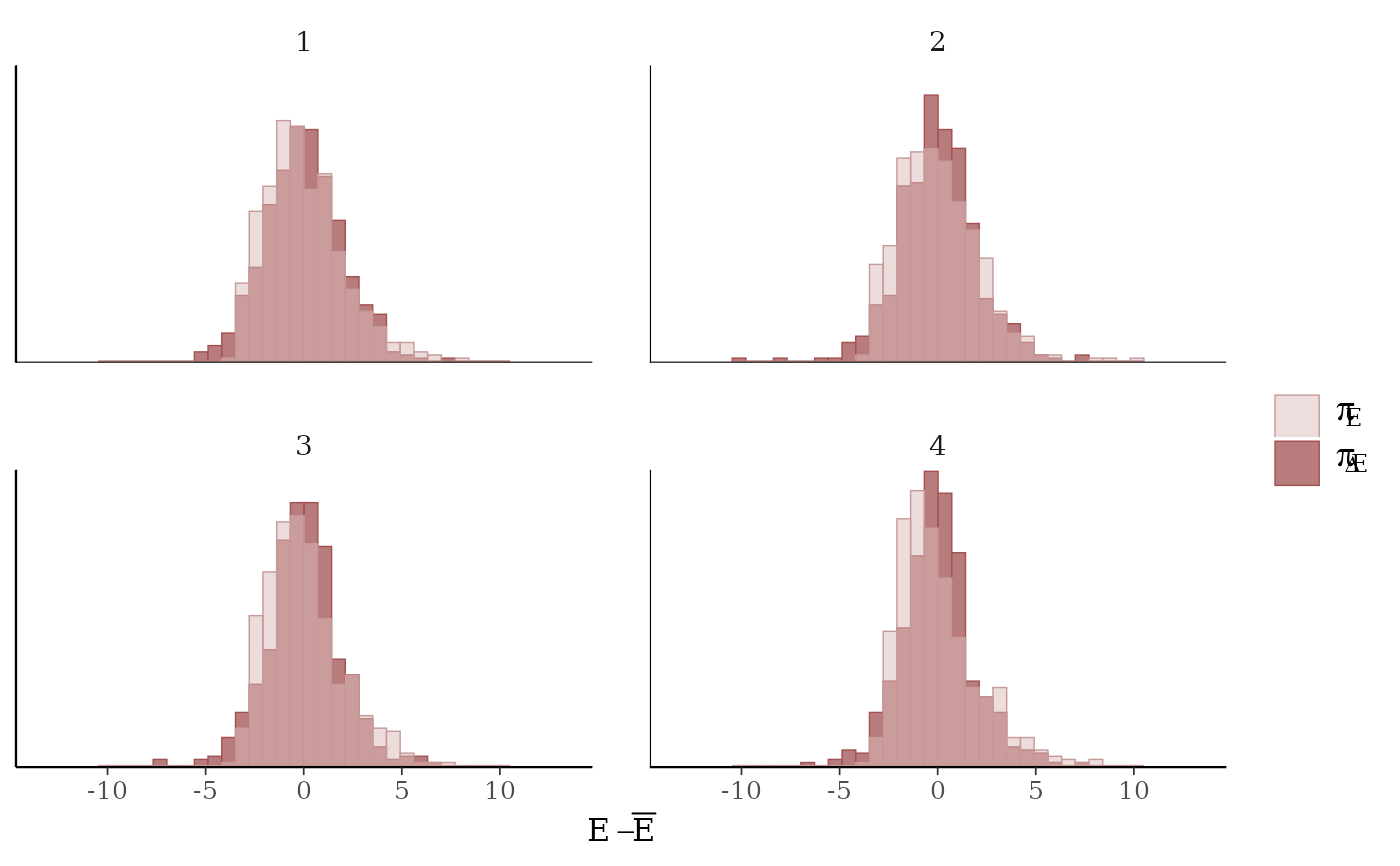mcmc_nuts_energy(np) +
facet_wrap(~ Chain, nrow = 1) +
coord_fixed(ratio = 150) +
ggtitle("NUTS Energy Diagnostic")#> stat_bin() using bins = 30. Pick better value with binwidth.#> stat_bin() using bins = 30. Pick better value with binwidth.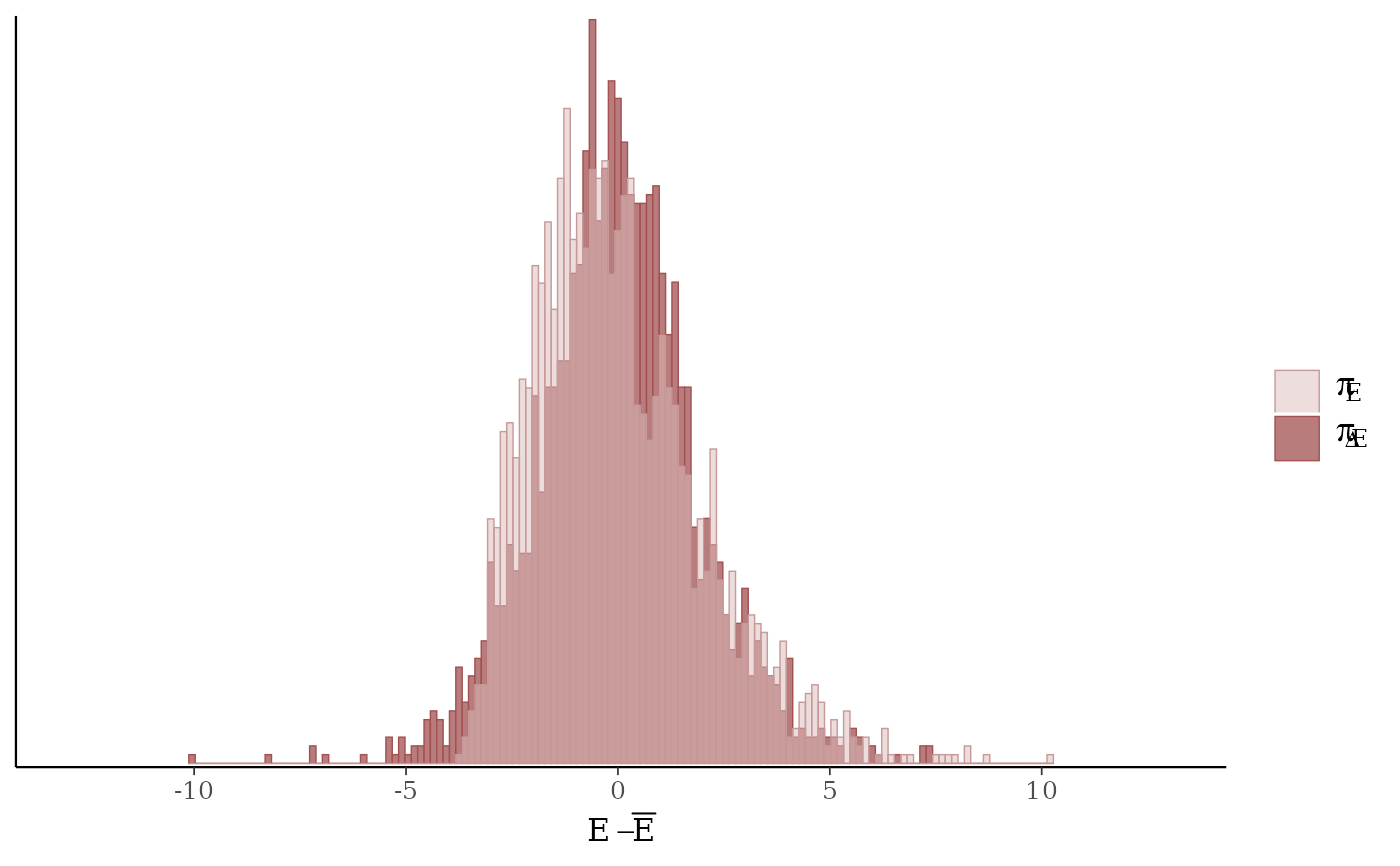# }